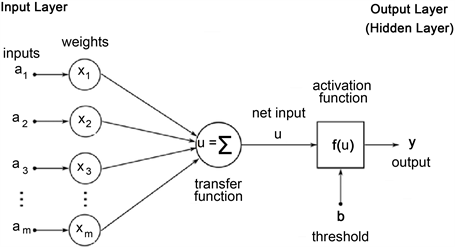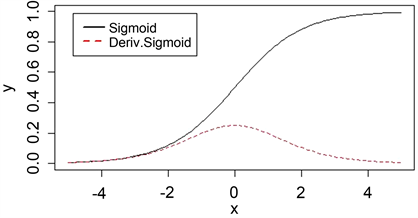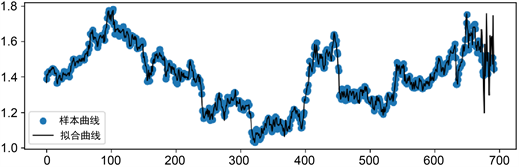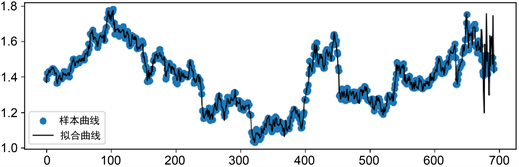﻿ 上证电信指数预测研究——基于人工神经网络模型

# 上证电信指数预测研究——基于人工神经网络模型Research on Shanghai Telecom Index Forecast—Based on Artificial Neural Network Model

Abstract: Artificial Neural Network has the strong ability of nonlinear dynamic processing problems, stock movements by many nonlinear factors, so this paper tries to use Python data analysis function, the use of artificial neural network model to forecast the Shanghai telecom index trend, and the prediction results compared with the actual result, judgment of the Artificial Neural Network in stock forecasting accuracy.

1. 引言

2. 国内研究现状

3. 人工神经网络

3.1. 算法原理Figure 1. ANN learning process computer algorithm for two-layer ANN with only input and output layers

$A=\left({a}_{1}\text{\hspace{0.17em}}\text{\hspace{0.17em}}\cdots \text{\hspace{0.17em}}\text{\hspace{0.17em}}{a}_{n}\right)=\left(\begin{array}{ccc}{a}_{11}& \cdots & {a}_{1n}\\ ⋮& \ddots & ⋮\\ {a}_{m1}& \cdots & {a}_{mn}\end{array}\right)$ (1)

$Y=\left(\begin{array}{c}{y}_{1}\\ ⋮\\ {y}_{m}\end{array}\right)$ (2)

$\left\{\begin{array}{l}{a}_{11}{x}_{1}+{a}_{12}{x}_{2}+\cdots +{a}_{1n}{x}_{n}+h={y}_{1}\\ {a}_{21}{x}_{1}+{a}_{22}{x}_{2}+\cdots +{a}_{2n}{x}_{n}+h={y}_{2}\\ \text{\hspace{0.17em}}\text{\hspace{0.17em}}\text{\hspace{0.17em}}\text{\hspace{0.17em}}\text{\hspace{0.17em}}\text{\hspace{0.17em}}\text{\hspace{0.17em}}\text{\hspace{0.17em}}\text{\hspace{0.17em}}\text{\hspace{0.17em}}\text{\hspace{0.17em}}\text{\hspace{0.17em}}\text{\hspace{0.17em}}\text{\hspace{0.17em}}\text{\hspace{0.17em}}\text{\hspace{0.17em}}\text{\hspace{0.17em}}\text{ }\text{\hspace{0.17em}}\text{\hspace{0.17em}}\text{\hspace{0.17em}}⋮\\ {a}_{m1}{x}_{1}+{a}_{m2}{x}_{2}+\cdots +{a}_{mn}{x}_{n}+h={y}_{m}\end{array}$ (3)

${A}_{mn}{X}_{n1}+{I}_{m1}h={Y}_{m1}$ (4)

$f\left({A}_{mn}{X}_{n1}+{I}_{m1}h\right)={Y}_{m1}$ (5)

3.2. 激活函数

$f\left(x\right)=\frac{1}{1+{\text{e}}^{-x}}=\left\{\begin{array}{l}0\text{\hspace{0.17em}}\text{\hspace{0.17em}}\text{\hspace{0.17em}}\text{\hspace{0.17em}}\text{\hspace{0.17em}}x\to -\infty \\ \frac{1}{2}\text{\hspace{0.17em}}\text{\hspace{0.17em}}\text{\hspace{0.17em}}\text{ }\text{ }\text{\hspace{0.17em}}x\to 0\\ 1\text{\hspace{0.17em}}\text{\hspace{0.17em}}\text{\hspace{0.17em}}\text{\hspace{0.17em}}\text{\hspace{0.17em}}\text{ }x\to +\infty \end{array}$ (6)

${f}^{\prime }\left(x\right)=\frac{{\text{e}}^{-x}}{{\left(1+{\text{e}}^{-x}\right)}^{2}}$ (7)Figure 2. Sigmoid function and derivative graph

$M={\mathrm{max}}_{i=1,2,\cdots ,n}\left\{{y}_{1},{y}_{2},\cdots ,{y}_{i},\cdots ,{y}_{n},K\right\}$ (8)

${y}_{i}=\frac{{y}_{i}}{M},\left(i=1,2,\cdots ,n\right)$ (9)

${y}_{i}=M{y}_{i},\left(i=1,2,\cdots ,n\right)$ (10)

3.3. 求解过程

1) 正向方程求解逻辑。

${U}_{m1}={A}_{mn}{X}_{n1}+{I}_{m1}h$ (11)

$f\left({U}_{m1}\right)={Y}_{m1}$ (12)

${E}_{m1}={Y}_{m1}-f\left({U}_{m1}\right)$ (13)

${\mathrm{min}}_{{X}_{n1},h}\left\{{E}_{m1}={Y}_{m1}-f\left({A}_{mn}{X}_{n1}+{I}_{m1}h\right)\right\}$ (14)

2) 通过反向传播(Backward Propagation, BP)调解，求最优解。

${X}_{n1}={X}_{n1}+{\delta }_{n1}$ (15)

$h=h+\tau$ (16)

$f\left({A}_{mn}\left({X}_{n1}+{\delta }_{n1}\right)+{I}_{m1}\left(h+\tau \right)\right)=f\left({U}_{m1}+{A}_{mn}{\delta }_{n1}+{I}_{m1}\tau \right)={Y}_{m1}$ (17)

${\mathrm{min}}_{{X}_{n1},h,{\delta }_{n1},\tau }\left\{{E}_{m1}={Y}_{m1}-f\left({A}_{mn}{X}_{n1}+{I}_{m1}h+{A}_{mn}{\delta }_{n1}+{I}_{m1}\tau \right)\right\}$ (18)

$f\left({U}_{m1}+{A}_{mn}{\delta }_{n1}+{I}_{m1}\tau \right)=f\left({U}_{m1}\right)+{f}^{\prime }\left({U}_{m1}\right)\left[{A}_{mn}{\delta }_{n1}+{I}_{m1}\tau \right]={Y}_{m1}$ (19)

${f}^{\prime }\left({U}_{m1}\right)\left[{A}_{mn}{\delta }_{n1}+{I}_{m1}\tau \right]={Y}_{m1}-f\left({U}_{m1}\right)={E}_{m1}$ (20)

3) 调节变量 ${\delta }_{n1}$$\tau$ 取值的确定

${A}_{mn}{\delta }_{n1}+{I}_{m1}\tau =\frac{{E}_{m1}}{{f}^{\prime }\left({U}_{m1}\right)}$ (21)

${A}_{mn}{\delta }_{n1}=\frac{{E}_{m1}}{{f}^{\prime }\left({U}_{m1}\right)}$ (22)

${A}_{mn}^{\text{T}}{A}_{mn}{\delta }_{n1}={A}_{mn}^{\text{T}}\left[\frac{{E}_{m1}}{{f}^{\prime }\left({U}_{m1}\right)}\right]$ (23)

${\left({A}_{mn}^{\text{T}}{A}_{mn}\right)}^{-1}{A}_{mn}^{\text{T}}{A}_{mn}{\delta }_{n1}={\left({A}_{mn}^{\text{T}}{A}_{mn}\right)}^{-1}{A}_{mn}^{\text{T}}\left[\frac{{E}_{m1}}{{f}^{\prime }\left({U}_{m1}\right)}\right]$ (24)

${\delta }_{n1}={\left({A}_{mn}^{\text{T}}{A}_{mn}\right)}^{-1}{A}_{mn}^{\text{T}}\left[\frac{{E}_{m1}}{{f}^{\prime }\left({U}_{m1}\right)}\right]$ (25)

${I}_{m1}\tau =\frac{{E}_{m1}}{{f}^{\prime }\left({U}_{m1}\right)}-{A}_{mn}{\delta }_{n1}$ (26)

${I}_{m1}^{\text{T}}{I}_{m1}\tau ={I}_{m1}^{\text{T}}\left\{\frac{{E}_{m1}}{{f}^{\prime }\left({U}_{m1}\right)}-{A}_{mn}{\delta }_{n1}\right\}$ (27)

${\left({I}_{m1}^{\text{T}}{I}_{m1}\right)}^{-1}{I}_{m1}^{\text{T}}{I}_{m1}\tau ={\left({I}_{m1}^{\text{T}}{I}_{m1}\right)}^{-1}{I}_{m1}^{\text{T}}\left\{\frac{{E}_{m1}}{{f}^{\prime }\left({U}_{m1}\right)}-{A}_{mn}{\delta }_{n1}\right\}$ (28)

$\tau ={\left({I}_{m1}^{\text{T}}{I}_{m1}\right)}^{-1}{I}_{m1}^{\text{T}}\left\{\frac{{E}_{m1}}{{f}^{\prime }\left({U}_{m1}\right)}-{A}_{mn}{\delta }_{n1}\right\}$ (29)

3.4. 利用二层人工神经网络算法进行股票指数预测

$S=\left\{{y}_{1},{y}_{2},\cdots ,{y}_{n-1},{y}_{n},{y}_{n+1},\cdots ,{y}_{{n}_{0}},{y}_{{n}_{0}+1},\cdots ,{y}_{{N}_{1}}\right\}$ (30)

${y}_{1+i}{x}_{1}+{y}_{2+i}{x}_{2}+\cdots +{y}_{n-1+i}{x}_{n-1}+h={y}_{n+i}$ (31)

$f\left({A}_{mn}{X}_{n1}+{I}_{m1}h\right)={Y}_{m1}$ (32)Figure 3. Schematic diagram of the selection of variables in the sample window of the ANN prediction program

4. 数据处理与预测结果

4.1. 数据的获取与假设

${B}_{t}=\frac{{A}_{1}}{{A}_{1}}\cdot \frac{{A}_{t}}{{A}_{t-1}}$ (33)

${A}_{t}$ ——第t天的上证电信指数；

${B}_{t}$ ——第t天的上证电信增长指数。

4.2. 参数含义Table 1. Goodness of fit between index forecast and growth index forecast

4.3. 预测结果分析Figure 4. Fitting results of the predicted value of Shanghai Telecom Index in the prediction periodFigure 5. Fitting results of the forecast value of the growth index of Shanghai Telecom in the forecast period

4.4. 预测实际检验

$Tren{d}_{t}=\left(\frac{{y}_{t}}{{y}_{t-1}}-1\right)\left(\frac{{\stackrel{^}{y}}_{t}}{{\stackrel{^}{y}}_{t-1}}-1\right)>0$ (34)

$Same\text{\hspace{0.17em}}Trend=\frac{Positive}{{N}_{1}-{n}_{0}}×100%$ (35)

$Different\text{\hspace{0.17em}}Trend=\frac{Negative}{{N}_{1}-{n}_{0}}×100%$ (36)Table 2. Actual forecast test of Shanghai Telecom Index and growth index

Table 3. Forecast results of Shanghai Telecom Index and growth index

5. 结论

 朱顺泉, 李一智. 基于人工神经网络BP算法的公司债券财务质量评级[J]. 计算机工程与应用, 2002(10): 243-245.

 孔维娜, 李跃进, 李双异, 裴久渤, 汪景宽. 人工神经网络产量定量评价模型在县域耕地地力评价中的应用[J]. 国土与自然资源研究, 2012(2): 30-32.

 贺昌政, 俞海. BP人工神经网络主成分分析预测模型及应用[J]. 数量经济技术经济研究, 2001(9): 104-106.

 赵瑞莹, 杨学成. 农产品价格风险预警模型的建立与应用——基于BP人工神经网络[J]. 农业现代化研究, 2008(2): 172-175.

 于佳. 房地产行业风险预警模型的应用研究[D]: [硕士学位论文]. 哈尔滨: 哈尔滨工业大学, 2006.

 彭涛. 人工神经网络房地产价格评估模型的研究及应用[D]: [硕士学位论文]. 西安: 长安大学, 2009.

 高琴. 人工神经网络在股市预测模型中的应用[J]. 微电子学与计算机, 2007(11): 147-151.

 汤江龙. 土地利用规划人工神经网络模型构建及应用研究[D]: [博士学位论文]. 南京: 南京农业大学, 2006.

 胡静. 神经网络在股票市场预测中的应用研究[D]: [硕士学位论文]. 济南: 山东师范大学, 2007.

 高攀. 人工神经网络模型在住宅类二手房价格评估中的应用研究[D]: [硕士学位论文]. 重庆: 重庆理工大学, 2017.

 阎虎勤. Python财务数据分析(讲义) [Z]. 厦门: 厦门国家会计学院, 2020.

Top